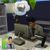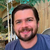# Discussion on: How can I generate random numbers in JavaScript?Hi! The way I do it is by using the "random" function on the built-in Math object. There are a bunch of great examples on MDN, but in a nutshell:

``````Math.random()  // will generate a random number between zero and one
Math.random() * x // will generate a random number between zero and x
Math.ceil(Math.random() * x) // will genearte a random integer between zero and x
``````Aaron McCollum

This ^^^

Also, you can write (Math.random() * x) + 1 to generate a number between 1 and x.